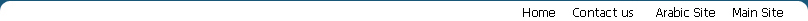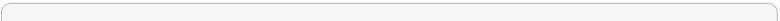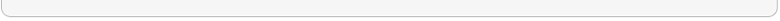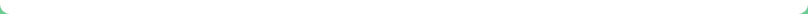About Services Portfolio Clients PartnersBFG D2D In Action Some Text is here Some Text is here Some Text is here Some Text is here Some Text is here Some Text is here Some Text is here Some Text is here Some Text is here Some Text is here Some Text is here Some Text is here Some Text is here Some Text is here Some Text is here Some Text is here Some Text is here Some Text is here Some Text is here Some Text is here Some Text is here Some Text is here Some Text is here Some Text is here Some Text is here Some Text is here Some Text is here Some Text is here Some Text is here Some Text is here Some Text is here Some Text is here Some Text is here Some Text is here Some Text is here Some Text is here Some Text is here Some Text is here Some Text is here Some Text is here Some Text is here Some Text is here Some Text is here Some Text is here Some Text is here Some Text is here Some Text is here Some Text is here Some Text is here Some Text is here Some Text is here Some Text is here Some Text is here Some Text is here Some Text is here Some Text is here Some Text is here Some Text is here Some Text is here Some Text is here Some Text is here Some Text is here Some Text is here Some Text is here Some Text is here Some Text is here Some Text is here Some Text is here Some Text is here Some Text is here Some Text is here Some Text is here Some Text is here Some Text is here Some Text is here Some Text is here Some Text is here Some Text is here Some Text is here Some Text is here Some Text is here Some Text is here Some Text is here Some Text is here Some Text is here Some Text is here Some Text is here Some Text is here Some Text is here Some Text is here Some Text is here Some Text is here Some Text is here Some Text is here Some Text is here Some Text is here Some Text is here Some Text is here Some Text is here Some Text is here Some Text is here Some Text is hereHome | About us | Services | Contact us Allright Reserved for BFG Door to Door 2010